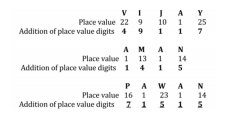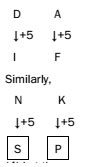# RRB ALP 2018 Practice Test Papers | Reasoning Questions (Day-23)

Dear Aspirants, Here we have given the Important RRB ALP & Technicians Exam 2018 Practice Test Papers. Candidates those who are preparing for RRB ALP 2018 can practice these Reasoning Questions to get more confidence to Crack RRB 2018 Examination.

[WpProQuiz 1815]

Click “Start Quiz” to attend these Questions and view Solutions

1. Choose the odd one out.

(A) Mumbai

(B) Kanpur

(C) Itanagar

(D) Dispur

1. Choose the number, which is different from the rest.

(A) 132

(B) 37

(C) 148

(D) 63

1. ZYXWVU, YXWVUZ,?, WVUZYX, VUZYXW

(A) XWVUZY

(B) XWVUYZ

(C) XWUVZY

(D) XVWUZY

1. In a certain code, ‘VIJAY’ is written as ‘49117’ and ‘AMAN’ is written as ‘1415’. How is ‘PAWAN’ written in the same code?

(A) 71155

(B) 71515

(C) 17515

(D) 71551

1. M and N are sisters, P is the brother of Q and the father of M. What is Q to N?

(A) Mother

(B) Brother

(C) None of these

(D) Cannot be determined

1. If ‘+’ means ‘divided by’, ‘–’ means ‘added to’, ‘*’ means ‘subtracted from’, and ‘/’ means ‘multiplied by’, then 26 – 15 + 5 × 4/ 2 =?

(A) 15

(B) 20

(C) 25

(D) None of the above

1. If ‘DA’ is related to ‘IF’, ‘NK’ will be related to

(A) SP

(B) PS

(C) PR

(D) SR

1. Insert the missing number. 1, 2, 2, 4, 2, 4, 2, 4, 6 ___

(A) 1

(B) 2

(C) 4

(D) 6

1. Insert the missing number. 0, 4, 18, 48, 100, ___

(A) 132

(B) 150

(C) 162

(D) 180

1. Insert the missing number. 64, 32, 48, 120, 420, ___

(A) 1890

(B) 1120

(C) 1240

(D) 720

Except Kanpur, all others are capitals of Indian states.

Among all the options, only 37 is a prime number.

In the given series, the next term is found by moving the first letter at the end of the term.  The required term is XWVUZY.5. Answer: (C)

Clearly, P is the father of M and N. It is also given that P is the brother of Q but the gender of Q is not given. Therefore Q is uncle or aunt of N.

If we put with correct symbols, the equation “26 – 15 + 5 * 4/ 2” would be written as

“26 + 15/ 5 – 4 × 2”. = 26 + 3 – 4 × 2 = 26 + 3 – 8 = 218. Answer: (B)

Each number in the given series is obtained by taking the difference of consecutive prime numbers. The pattern of the series is as follows: 3 – 2 = 1

5 – 3 = 2

7 – 5 = 2

11 – 7 = 4

13 – 11 = 2

17 – 13 = 4

19 – 17 = 2

23 – 19 = 4

29 – 23 = 6

The next number = 31 – 29 = 2

The pattern of the series is as follows: 13 – 12 = 0

23 – 22 = 4

33 – 32 = 18

43 – 42 = 48

53 – 52 = 100

The next number = 63 – 62 = 180

The pattern of the series is as follows:

64 × 0.5 = 32

32 × 1.5 = 48

48 × 2.5 = 120

120 × 3.5 = 420

The next number = 420 × 4.5 = 1890

RRB ALP 2018 Practice Test Papers | Reasoning Questions (Day-1)

RRB ALP 2018 Practice Test Papers | Reasoning Questions (Day-2)

RRB ALP 2018 Practice Test Papers | Reasoning Questions (Day-3)

RRB ALP 2018 Practice Test Papers | Reasoning Questions (Day-4)

RRB ALP 2018 Practice Test Papers | Reasoning Questions (Day-5)

RRB ALP 2018 Practice Test Papers | Reasoning Questions (Day-6)

RRB ALP 2018 Practice Test Papers | Reasoning Questions (Day-7)

RRB ALP 2018 Practice Test Papers | Reasoning Questions (Day-8)

RRB ALP 2018 Practice Test Papers | Reasoning Questions (Day-9)

RRB ALP 2018 Practice Test Papers | Reasoning Questions (Day-10)

RRB ALP 2018 Practice Test Papers | Reasoning Questions (Day-11)

RRB ALP 2018 Practice Test Papers | Reasoning Questions (Day-12)

RRB ALP 2018 Practice Test Papers | Reasoning Questions (Day-13)

RRB ALP 2018 Practice Test Papers | Reasoning Questions (Day-14)

RRB ALP 2018 Practice Test Papers | Reasoning Questions (Day-15)

RRB ALP 2018 Practice Test Papers | Reasoning Questions (Day-16)

RRB ALP 2018 Practice Test Papers | Reasoning Questions (Day-17)

RRB ALP 2018 Practice Test Papers | Reasoning Questions (Day-18)

RRB ALP 2018 Practice Test Papers | Reasoning Questions (Day-19)

RRB ALP 2018 Practice Test Papers | Reasoning Questions (Day-20)

RRB ALP 2018 Practice Test Papers | Reasoning Questions (Day-21)

RRB ALP 2018 Practice Test Papers | Reasoning Questions (Day-22)